# 2.4 Wallpaper Groups

A group of symmetries of the plane that is doubly infinite is a crystallographic group, or wallpaper group. There are 17 types of such groups, corresponding to 17 essentially distinct ways to tile the plane in a doubly periodical pattern.

The simplest wallpaper group involves translations only (, left). The others involve, in addition to translations, one or more of the other types of symmetries (rotations, reflections, glide-reflections). The Conway notation for wallpaper groups is based on what types of non-translational symmetries occur in the ``simplest description'' of the group: * indicates a reflection (mirror symmetry), × a glide-reflection, and a number n indicates a rotational symmetry of order n (rotation by 360°/n). In addition, if a number n comes after the *, the center of the corresponding rotation lies on mirror lines, so the symmetry there is actually dihedral of order 2n.

Thus the ** grouphas two inequivalent lines of mirror symmetry; the 333 grouphas three inequivalent centers of order-3 rotation; the 22* grouphas two inequivalent centers of order-2 rotation as well as mirror lines; and 632has points of dihedral symmetry of order 12(=2×6), 6, and 4.

The following table gives the groups in the Conway notation and in the notation traditional in crystallography. It also gives the quotient space of the plane by the action of the group. The entry ``4,4,2 turnover'' means the surface of a triangular puff pastry with corner angles 45°(=180°/4), 45° and 90°. The entry ``4,4,2 turnover slit along 2,4'' means the same surface, slit along the edge joining a 45° vertex to the 90°vertex. Open edges are silvered (mirror lines); such edges occur exactly for those groups whose Conway notation includes a *.

The last column of the table gives the number of degrees of freedom in the choice of the group, that is, the dimension of the space of inequivalent groups of the given type (equivalent groups are those that can be obtained from one another by proportional scaling or rigid motion). For instance, there is a group of typefor every shape parallelogram, and there are two degrees of freedom for the choice of such a shape (say ratio and angle between sides). Thus, thegroup shown hereis based on a square fundamental domain, while for thegroup shown herea fundamental parallelogram would have the shape of two juxtaposed equilaterateral triangles. These two groups are inequivalent, although they are of the same type.

[NOTE: For readers whose browser does not support tables, all this information is repeated below together with the figures.]

 figure Conway cryst quotient space deg, left, leftp1 torus 2, right ×× pg Klein bottle 1, left ** pm cylinder 1, right ×* cm Möbius strip 1, left 22× pgg nonorientable football 1, right 22* pmg open pillowcase 1, left 2222 p2 closed pillowcase 2, right 2*22 cmm 4,4,2 turnover, slit along 4,4 1, left *2222 pmm square 1, right 442 p4 4,4,2 turnover 0, left 4*2 p4g 4,4,2 turnover, slit along 4,2 0, right *442 p4m 4,4,2 triangle 0, right 333 p3 3,3,3 turnover 0, left *333 3m1 3,3,3 triangle 0, right 3*3 p31m 6,3,2 turnover, slit along 3,2 0, left 632 p6 6,3,2 turnover 0, right *632 p6m 6,3,2 triangle 0

The figures below show wallpaper patterns based on each of the 17 types of crystallographic groups (two patterns are shown for the, or translations-only, type). Thin lines bound unit cells, or fundamental domains. When solid, they represent lines of mirror symmetry, and are fully determined. When dashed, they represent arbitrary boundaries, which can be shifted so as to give different fundamental domain. One can even make these lines into curves, provided the symmetry is respected. Dots at the intersections of thin lines represent centers of rotational symmetry.

Some of the relationships between the types are made obvious by the patterns. For instance, of the groups here, we see that the group on the right, of type ××, contains the one on the left, of type, with index two. However, there are more relationships than can be indicated in a single set of pictures: for instance, there is a group of type ×× hiding in any group of type 3*3.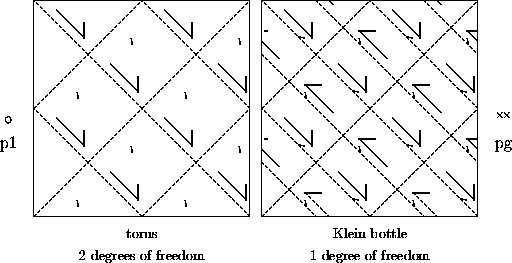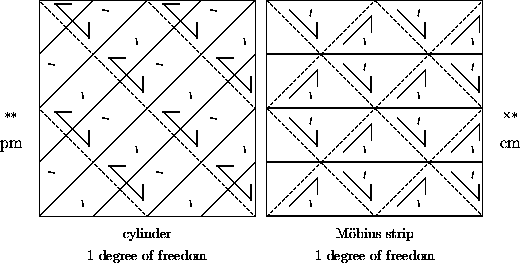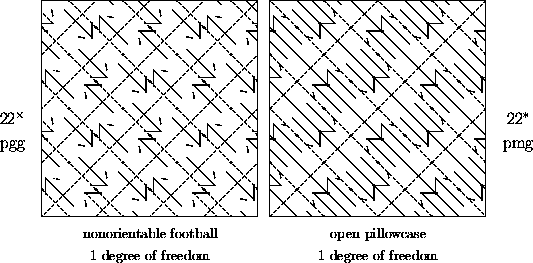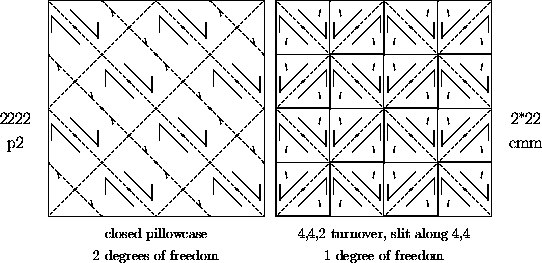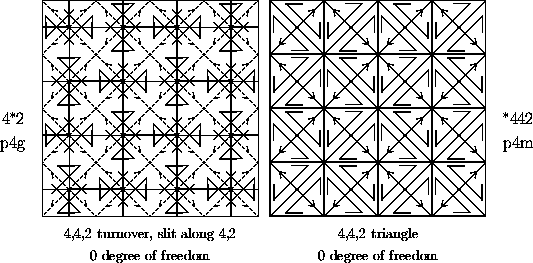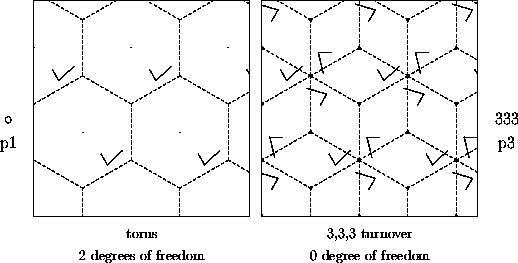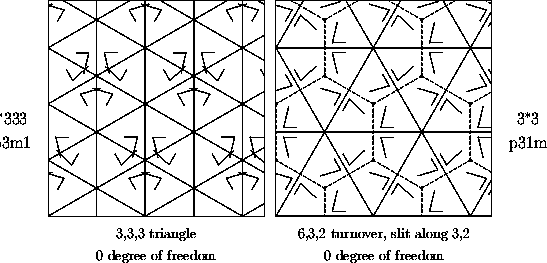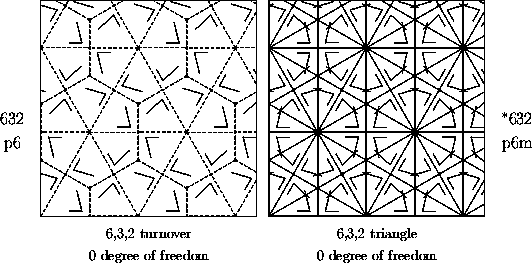Next: 3 Other Transformations of the Plane
Up: 2 Plane Symmetries or Isometries
Previous: 2.3 Formulas for Symmetries in Polar CoordinatesThe Geometry Center Home Page

Silvio Levy
Wed Oct 4 16:41:25 PDT 1995

This document is excerpted from the 30th Edition of the CRC Standard Mathematical Tables and Formulas (CRC Press). Unauthorized duplication is forbidden.